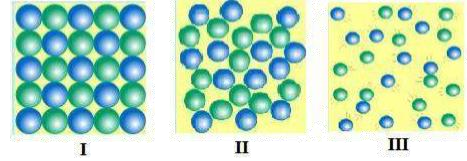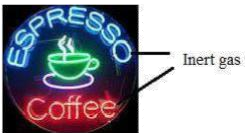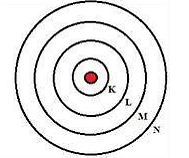### ICSE 8th Chemistry Sample Paper-2# ICSE CLASS 8 CHEMISTRY SAMPLE PAPER – 2

CHEMISTRY – VIII

Paper – 1 (THEORY)

Time: 3 Hours

General Instructions:

1. All questions are compulsory.

2. Questions 1 to 15 carry 1 mark each.

3. Questions in 2A and 2B carry 1 mark each.

4. Questions in 3A and 3B carry 1 mark each.

5. Question 4A and 4B carry 5 marks each.

6. Question 5A and 5B carry 5 marks each.

7. Question 6A and 6B carry 5 marks each.

8. Question 7A and 7B carry 5 marks each.

# Question 1  (1 mark each)

Choose the correct answer out of the four available choices given under each question.

1. Which of the following is incorrect about a heterogeneous mixture?

(a) Constituents can be distinctly seen

(b) Constituents are uniformly mixed

(c) Different composition throughout its mass

(d) Different composition in different parts of its mass

2. The valency of carbon is 4 and that of oxygen is 2. What is the molecular formula of carbon dioxide?

(a) $mathrm{C}_{2} mathrm{O}$                (b) $mathrm{C}_{2} mathrm{O}_{2}$

(c) CO                (d) $mathrm{CO}_{2}$

3. Which of the following pictures represents the arrangement of particles in liquids?(a) Picture II               (b) Picture I and II

(c) Picture I              (d) Picture II and III

4. Which of the following are combination reactions?

i. $4 mathrm{~K}+mathrm{O}_{2} rightarrow 2 mathrm{~K}_{2} mathrm{O}$

ii. $mathrm{CuSO}_{4}+2 mathrm{NH}_{4} mathrm{OH} rightarrowleft(mathrm{NH}_{4}right) 2 mathrm{SO}_{4}+mathrm{Cu}(mathrm{OH})_{2}$

iii. $2 mathrm{ZnS}+3 mathrm{O}_{2} rightarrow 2 mathrm{ZnO}+2 mathrm{SO}_{2}$

iv. $mathrm{Na}_{2} mathrm{O}+mathrm{H}_{2} mathrm{O} rightarrow 2 mathrm{NaOH}$

(a) Reactions (i) and (ii)                     (b) Reactions (ii) and (iii)

(c) Reactions (ii) and (iv)                 (d) Reactions (i) and (iv)

5. If atomic number of an atom is 17 and mass number is 35 then number of neutrons will be

(a) 35               (b) 17

(c) 18              (d) 52

6. Valency of iron in FeO is _____ of chlorine in $mathrm{CaCl}_{2}$ is_________.

(a) 1+, 2 (b) 2+, 1

(c) 2+, 2               (d) 1+, 1

7. The Element __________ has symbol derived from its Latin name ‘plumbum’.

(c) Carbon  (d) Hydrogen

8. Which inert gas is used in the advertisement hoarding shown in the given picture?(c) Krypton              (d) Neon

9. When the temperature of water increases above $0^{circ} mathrm{C}$ up to $4^{circ} mathrm{C}$, its density ________.

(a) Decreases                    (b) Increases

(c) Becomes zero              (d) Remains unchanged

10. In metal reactivity series the most reactive metal are at

(a) Top                      (b) Bottom

(c) Middle                 (d) None

11. When an electric current is passed through acidulated water, volume of hydrogen is formed at the cathode and ______ volume of oxygen is formed at the anode.

(a) one, two                 (b) three, one

(c) one, three              (d) two, one

12. The process of removing oxygen from their compounds is called _______.

(a) Reduction              (b) Combination

(c) Synthesis                (d) Oxidation

13. A soluble solid is separated from insoluble solid by _________

(a) Fractional crystallisation  (b) Solvent extraction

(c) Sublimation              (d) Magnetic separation.

14. The ________ of water enables aquatic animals to survive in a reservoir where the surface layer of water has frozen.

(a) Anomalous expansion  (b) Anomalous contraction lead

(c) Volume            (d) Temperature

15. Which of the following shells represent the orbit number (n) = 2?(a) K shell                 (b) L shell

(c) M shell              (d) N shell

# Question 2  (5 mark each)

(A) Define Matter. State the main postulates of kinetic theory of matter.

(B) Fill in the blanks and rewrite the sentences:

1. The gas which has now replaced hydrogen in air balloons is _____.

2. The crystal of _______ is opaque to light and is good conductor of heat.

3. Water reacts with metals to liberate ________ gas.

4. The process of change from the ________ state to the ________ state at a particular temperature is called liquefaction.

5. Atoms of the same elements differing in the number of __________ in their nuclei are known as isotopes.

# Question 3  (5 mark each)

(A) State whether the following statements are true or false.

Rewrite the false statement.

2. Carbon monoxide is a poisonous gas.

3. The rays emitted from the cathode towards the anode in the discharge tube are called cathode rays.

4. Distilled water is used as an electrolyte in the electrolysis of water.

5. Graphite is the purest form of carbon.

(B) Explain efflorescence and deliquescence with the help of an example.

# Question 4  (5 mark each)

(A) Describe the formation of coal. What are its four types?

(B) State physical properties of water. How does anomalous expansion of water help aquatic

organism in cold climates?

# Question 5  (5 mark each)

(A) What is atom? State the main postulates of Dalton’s atomic theory.

(B) Draw a neat labelled diagram for the laboratory preparation of hydrogen. Give balanced equations for reaction and how is hydrogen gas is collected? Why?

# Question 6  (5 mark each)

(A) Explain with example:

1. Displacement reaction

2. Double displacement reaction

3. Neutralisation reaction.

4. Combination reaction

5. Decomposition reaction

(B) State the atomic number, mass number and electronic configuration for each of the following

elements:

1. Magnesium [p = 12, n = 12]

2. Boron [p = 5, n = 6]

3. Sodium [p = 11, n = 12]

4. Carbon [p = 6, n = 6]

5. Helium [p = 2, n = 2]

# Question 7  (5 mark each)

(A)  1. Differentiate between physical change and chemical change

2. State the formula of the following compounds:

(a) Ammonium bicarbonate      (b) Aluminum oxide

(B)  1. Explain the term compound with suitable examples.

2. What is destructive distillation? What are the products formed due to the destructive distillation of coal?

Privacy Settings